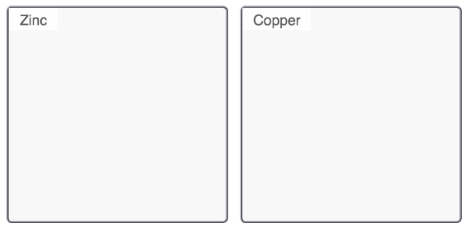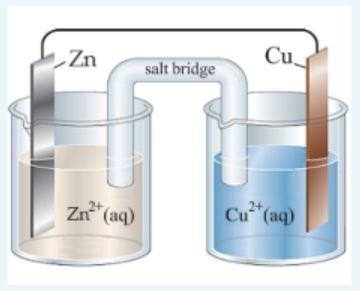# Problem: Part ADescribe the electrodes in this zinc-copper galvanic cell. Drag the appropriate items to their respective bins.anode      cathode      gains mass      loses massPart BZn(s) + Cu2+ (aq) → Zn2+ (aq) + Cu(s)Consider the following: Zn2+ (aq) + 2e- → Zn(s),      E° red = -0.763 VCu2+ (aq) + 2e- → Cu(s),     E° red = +0.337 VWhat is the standard potential, Ecell, for this galvanic cell? Use the given standard reduction potentials in your calculation as appropriate. Express your answer to three decimal places and include the appropriate units.

###### FREE Expert Solution

Part A

Zn(s) + Cu2+(aq) → Zn2+(aq) + Cu(s)

 Anode → oxidation

oxidation half-reaction happens in the anode solution
▪ losing electrons → becoming more positive → anode dissolves away

Zn(s) → Zn2+ (aq)

Zn → anode → loses mass

 Cathode → reduction

reduction half-reaction happens in the anode solution
▪ gaining electrons → becoming more negative → solids is deposited in the surface

Cu2+(aq) → Cu(s)

Cu → cathode → gains mass

Part B

98% (318 ratings)###### Problem Details

Part A

Describe the electrodes in this zinc-copper galvanic cell. Drag the appropriate items to their respective bins.

anode      cathode      gains mass      loses massPart B

Zn(s) + Cu2+ (aq) → Zn2+ (aq) + Cu(s)Consider the following:

Zn2+ (aq) + 2e- → Zn(s),      E° red = -0.763 V

Cu2+ (aq) + 2e- → Cu(s),     E° red = +0.337 V

What is the standard potential, Ecell, for this galvanic cell? Use the given standard reduction potentials in your calculation as appropriate. Express your answer to three decimal places and include the appropriate units.